# python数据分析：基于协同过滤的电影推荐算法

## 协同过滤

### 优缺点

#### 优点

• 能够过滤机器难以自动内容分析的信息，如艺术品，音乐等。
• 共享其他人的经验，避免了内容分析的不完全或不精确，并且能够基于一些复杂的，难以表述的概念（如信息质量、个人品味）进行过滤。
• 有推荐新信息的能力。可以发现内容上完全不相似的信息，用户对推荐信息的内容事先是预料不到的。可以发现用户潜在的但自己尚未发现的兴趣偏好。
• 推荐个性化、自动化程度高。能够有效的利用其他相似用户的反馈信息。加快个性化学习的速度。

#### 缺点

• 新用户问题(New User Problem) 系统开始时推荐质量较差
• 新项目问题(New Item Problem) 质量取决于历史数据集
• 稀疏性问题（Sparsity）
• 系统延伸性问题（Scalability）。

## python实现

### 数据导入

# 导入各种库
import pandas as pd
import numpy as np
import matplotlib.pyplot as plt
from scipy.sparse import csr_matrix
from sklearn.neighbors import NearestNeighbors
from sklearn.decomposition import TruncatedSVD

# 导入电影链接文件
links = pd.read_csv('links.csv')
links.head()

# 导入电影信息文件
movies = pd.read_csv('movies.csv')
movies.head()

# 导入评分文件
ratings = pd.read_csv('ratings.csv')
ratings.head()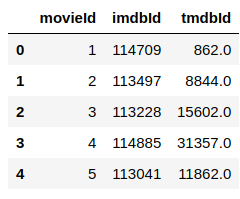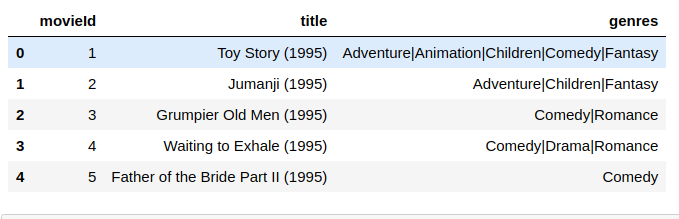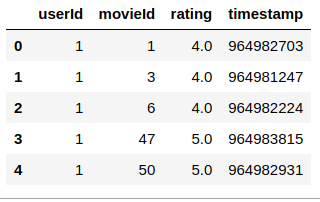### 构建电影详细信息

# 讲电影信息和电影链接根据电影id合并
movies_add_links = pd.merge(movies, links ,on='movieId')

def get_year(x):
try:
y = int(x.strip()[-5:-1])
except:
y = 0

return y
# 获取电影年份
movies_add_links['movie_year'] = movies_add_links['title'].apply(get_year)

# 计算每个电影的被评论次数
rating_counts = pd.DataFrame(ratings.groupby('movieId').count()['rating'])
rating_counts.rename(columns={'rating':'ratingCount'}, inplace=True)
# 合并到一起
movie_add_rating = pd.merge(movies_add_links, rating_counts, on='movieId')

# 获取每个电影的平均评分并合并
rating_means = pd.DataFrame(ratings.groupby('movieId').mean()['rating'])
rating_means.columns=['rating_mean']
movie_total = pd.merge(movie_add_rating, rating_means, on='movieId')
movie_total.head()


pandas更改列名可以使用rename进行精准更改，也可以世界对df.columns进行操作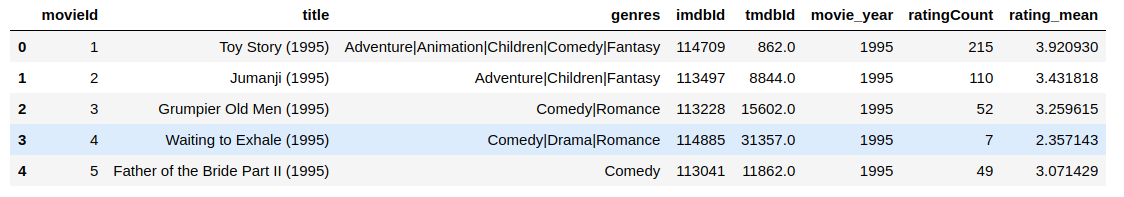### 简单分析数据

# 评分分布情况
ratings.rating.value_counts(sort=True).plot('bar')
plt.title('Rating Distribution\n')
plt.xlabel('Rating')
plt.ylabel('Count')
plt.savefig('rating.png', bbox_inches='tight')
plt.show()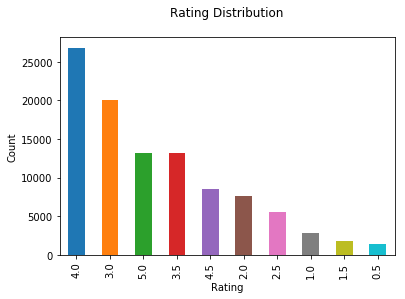### 可视化每年电影数量和每年电影被评论数量 ###
# 设置画幅
plt.figure(figsize=(16,16))
# 分层
plt.subplot(2,1,1)
# 每年电影数
movie_total.groupby('movie_year')['ratingCount'].count().plot('bar')
plt.title('Movies counts by years\n')
plt.xlabel('years')
plt.ylabel('counts')
# 每年电影评论数
plt.subplot(2,1,2)
movie_total.groupby('movie_year')['ratingCount'].sum().plot('bar')
plt.title('Movies ratings by years\n')
plt.xlabel('years')
plt.ylabel('ratings')
plt.savefig('mix.png', bbox_inches='tight')
plt.show()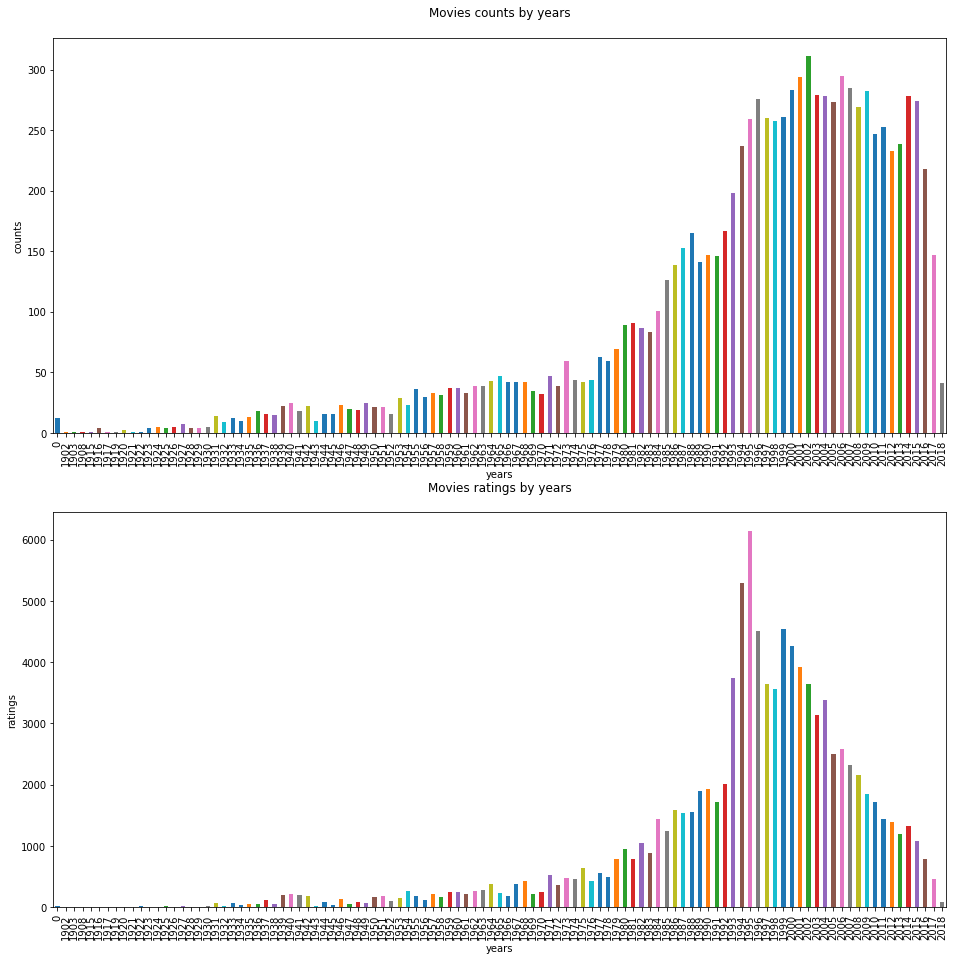• 可见1980年后电影数量飞速增长，到1995年平稳
• 虽然1995到2016年电影数量基本相差不是很大，但是1994,1995年电影的评论数（关注程度）远高于以后
• 猜测1995年为电影的鼎盛时期

### 构建用于筛选电影数据

# 构建要使用与筛选的电影信息
combine_movie = pd.merge(ratings, rating_counts, on='movieId')
combine_movie = combine_movie.dropna()
combine_movie.head()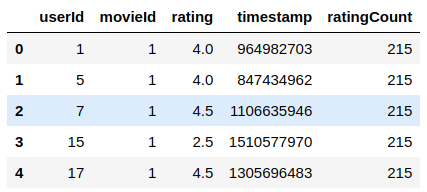# 计算70%评论数为多少用以筛选
combine_movie.ratingCount.quantile(np.arange(.5, 1, .05))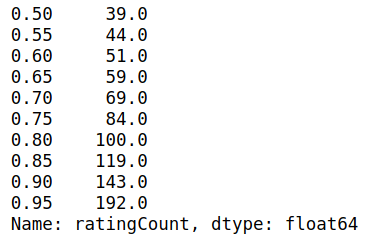popularity_threshold = 69
# 根据位置进行筛选
rating_popular_movie = combine_movie.query('ratingCount >= @popularity_threshold')
rating_popular_movie.head()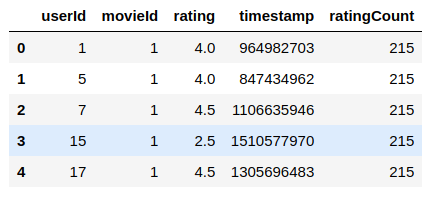# 讲表格转化为2D矩阵
movie_pivot = rating_popular_movie.pivot(index='movieId', columns='userId', values='rating').fillna(0)

# 看看用户多少，电影多少
movie_pivot.shape



(264, 602)
264个电影， 602个用户

# 选出评分数最多的电影id
movie_1 = ratings.groupby('movieId').count().sort_values('rating', ascending=False).index
movie_total[movie_total.movieId == movie_1]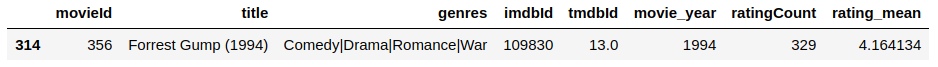### 推荐算法

#### 根据皮尔森关联度

# 获取电影详细信息函数
def get_movie(df_movie, moive_list):
# 根据电影ID获取电影详细信息
df_movieId = pd.DataFrame(movie_list, index=np.arange(len(movie_list)), columns=['movieId'])
corr_movies = pd.merge(df_movieId, movie_total, on='movieId')
return corr_movies

def person_method(df_movie, pivot, movie, num):
# 获取目标电影属性
bones_ratings = pivot[movie]
# 计算出电影跟该电影的皮尔森相关性
similar_to_bones = pivot.corrwith(bones_ratings)
corr_bones = pd.DataFrame(similar_to_bones, columns=['pearson'])
# 弃去缺失值
corr_bones.dropna(inplace=True)
# 相关性与评论数合并
corr_summary = corr_bones.join(df_movie[['movieId','ratingCount']].set_index('movieId'))
# 刷选出对应数量的高关联性电影
movie_list = corr_summary[corr_summary['ratingCount'] >= 100].sort_values('pearson', ascending=False).index[:num].tolist()
return movie_list

# 获取电影ID列表
movie_list = person_method(movie_total, movie_pivot, movie_1, 6)
# 获取电影详细数据
corr_movies = get_movie(movie_total, movie_list)
corr_movies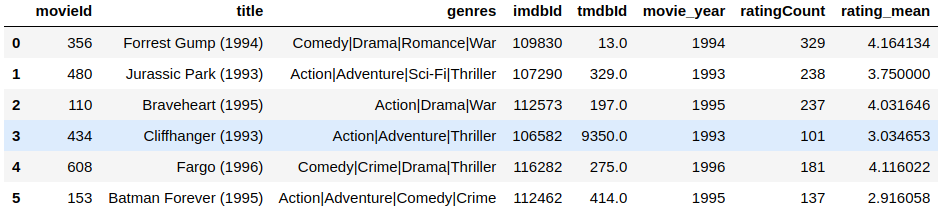#### 基于knn

def knn_method(movie_pivot, movie, num):
# 压缩稀疏矩阵
movie_pivot_matrix = csr_matrix(movie_pivot.values)
# 我们用来计算最近邻居的算法是“brute”，我们指定“metric =cosine”，以便算法计算评级向量之间的余弦相似度。
model_knn = NearestNeighbors(metric = 'cosine', algorithm = 'brute')
# 训练模型
model_knn.fit(movie_pivot_matrix)
# 根据模型找到与所选电影最近6个（包含其本身）电影
distances, indices = model_knn.kneighbors(movie_pivot.loc[[movie], :].values.reshape(1, -1), n_neighbors = num)
# 获取电影ID列表
movie_list = movie_pivot.iloc[indices,:].index
return movie_list

movie_list = knn_method(movie_pivot, movie_1, 6)
corr_movies = get_movie(movie_total, movie_list)
corr_movies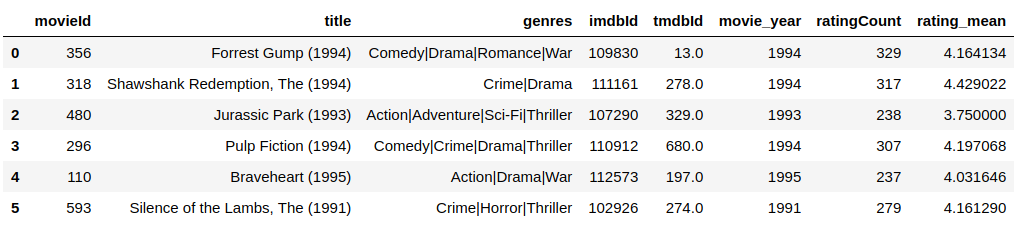#### 基于矩阵分解

def SVD_method(movie_pivot, movie, num):
# 使用SVD对信息进行矩阵分解
SVD = TruncatedSVD(n_components=12, random_state=17)
matrix = SVD.fit_transform(movie_pivot.values)
# 构建后信息df
movie_SVD = pd.DataFrame(matrix, index=movie_pivot.index).T
# 求电影之间皮尔森相关度
corr = movie_SVD.corr()
# 根据提供的电影查找相近的5个电影
search_movie = movie_pivot.loc[[movie], :].index
movie_list = corr.sort_values(search_movie, ascending=False).index[0:num].tolist()
return movie_list

movie_list = SVD_method(movie_pivot, movie_1, 6)
corr_movies = get_movie(movie_total, movie_list)
corr_movies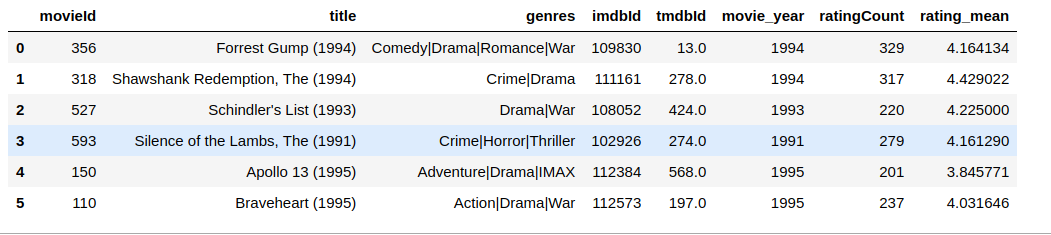### 总结07-084400
04-27
12-04440
08-131806
04-072315
12-111362
06-094944
©️2020 CSDN 皮肤主题: 酷酷鲨 设计师:CSDN官方博客